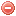# Definition

Precipitation duration in hours is used by 100-hr and 1000-hr fuel moisture indices of the NDRFS.
When absent it can be estimated usinga a formula proposed by Bradshaw et al. (1983).

# Formula

Bradshaw et al. (1983) proposed to replace the $$P_{Dur}$$ as follows:

$P_{Dur}= min( 8, \dfrac{P + 0.02)}{p_r})$

with the precipitation rate $$p_r$$ [inch/h] defined as

$p_r = \begin{cases} 0.25, & \mbox{for } Climate {\ 1\ or\ 2} \\ &\\ 0.05, & \mbox{for } Climate {\ 3\ or\ 4} \\ \end{cases}$

where $$P$$ is the 24-hours precipitation in inches and $$Climate$$ is the climate class according to Deeming (1977).

Literature:
Deeming (1977)

### Bibliography SearchNo results!

No results were found. Are you sure you searched for a tag?

### Symbols

 Variable Description Unit $$T$$ air temperature °C $$T_{dew}$$ dew point temperature °C $$H$$ air humidity % $$P$$ rainfall mm $$U$$ windspeed m/s $$w$$ days since last rain (or rain above threshold) d $$rr$$ days with consecutive rain d $$\Delta t$$ time increment d $$\Delta{e}$$ vapor pressure deficit kPa $$e_s$$ saturation vapor pressure kPa $$e_a$$ actual vapor pressure kPa $$p_{atm}$$ atmospheric pressure kPa $$PET$$ potential evapotranspiration mm/d $$r$$ soil water reserve mm $$r_s$$ surface water reserve mm $$EMC$$ equilibrium moisture content % $$DF$$ drought factor - $$N$$ daylight hours hr $$D$$ weighted 24-hr average moisture condition hr $$\omega$$ sunset hour angle rad $$\delta$$ solar declination rad $$\varphi$$ latitude rad $$Cc$$ cloud cover Okta $$J$$ day of the year (1..365/366) - $$I$$ heat index - $$R_n$$ net radiation MJ⋅m-2⋅d-1 $$R_a$$ daily extraterrestrial radiation MJ⋅m-2⋅d-1 $$R_s$$ solar radiation MJ⋅m-2⋅d-1 $$R_{so}$$ clear-sky solar radiation MJ⋅m-2⋅d-1 $$R_{ns}$$ net shortwave radiation MJ⋅m-2⋅d-1 $$R_{nl}$$ net longwave radiation MJ⋅m-2⋅d-1 $$\lambda$$ latent heat of vaporization MJ/kg $$z$$ elevation m a.s.l. $$d_r$$ inverse relative distance Earth-Sun - $$\alpha$$ albedo or canopy reflection coefficient - $$\Delta$$ slope of the saturation vapor pressure curve kPa/°C $$Cc$$ cloud cover eights $$ROS$$ rate of spread m/h $$RSF$$ rate of spread factor - $$WF$$ wind factor - $$WRF$$ water reserve factor - $$FH$$ false relative humidity - $$FAF$$ fuel availability factor - $$PC$$ phenological coefficient -

 Suffix Description $$-$$ mean / daily value $$_{max}$$ maximum value $$_{min}$$ minimum value $$_{12}$$ value at 12:00 $$_{13}$$ value at 13:00 $$_{15}$$ value at 15:00 $$_{m}$$ montly value $$_{y}$$ yearly value $$_{f/a}$$ value at fuel-atmosphere interface $$_{dur}$$ duration $$_{soil}$$ value at soil level

 Constant Description $$e$$ Euler's number $$\gamma$$ psychrometric constant $$G_{SC}$$ solar constant $$\sigma$$ Stefan-Bolzmann constant